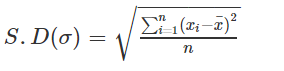# Properties of Standard Deviation

Statistics refers to the study of the analysis, interpretation, collection, presentation, and organization of data. Standard deviation is an important topic in statistics. It is the measure of dispersion of a set of data from its mean. It measures the absolute variability of a distribution. It shows a typical deviation from the mean. The formula for standard deviation is given by.

In this article, we will learn the important properties of standard deviation.

## 6 Important Properties of Standard Deviation

1. It cannot be negative.

2. It is only used to measure spread or dispersion around the mean of a data set.

3. It shows how much variation or dispersion exists from the average value.

4. It is sensitive to outliers. A single outlier can raise σ and in turn, distort the picture of spread.

5. For data with almost the same mean, the greater the spread, the greater the standard deviation.

6. Standard deviation can be used in conjunction with the mean in order to calculate data intervals when analyzing normally distributed data.

### Example

Find the standard deviation for the following data series:

10, 6, 7, 3, 15, 8, 2, 5.

Solution:

Given set of data = 10, 6, 7, 3, 15, 8, 2, 5.

Mean = (10+6+7+3+15+8+2+5)/8 = 7

Standard deviation = √[(10-7)2 +(6-7)2+ (7-7)2+ (3-7)2+(15-7)2+ (8-7)2+(2-7)2+(5-7)2/8]

= √(9+1+0+16+64+1+25+4)/8 = 3.872